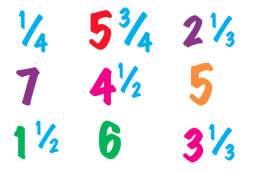# Subtract mixed 2

Subtract mixed numbers: 3 1/2 - 2 4/5 (3 and one half - 2 and four-fifths.) Remember you need to make these into improper fractions before subtracting.

x =  7/10

### Step-by-step explanation:Did you find an error or inaccuracy? Feel free to write us. Thank you!

Showing 1 comment:
Sogo
More details ... Inaccuracy

Tips for related online calculators
Need help calculating sum, simplifying, or multiplying fractions? Try our fraction calculator.
Need help with mixed numbers? Try our mixed-number calculator.